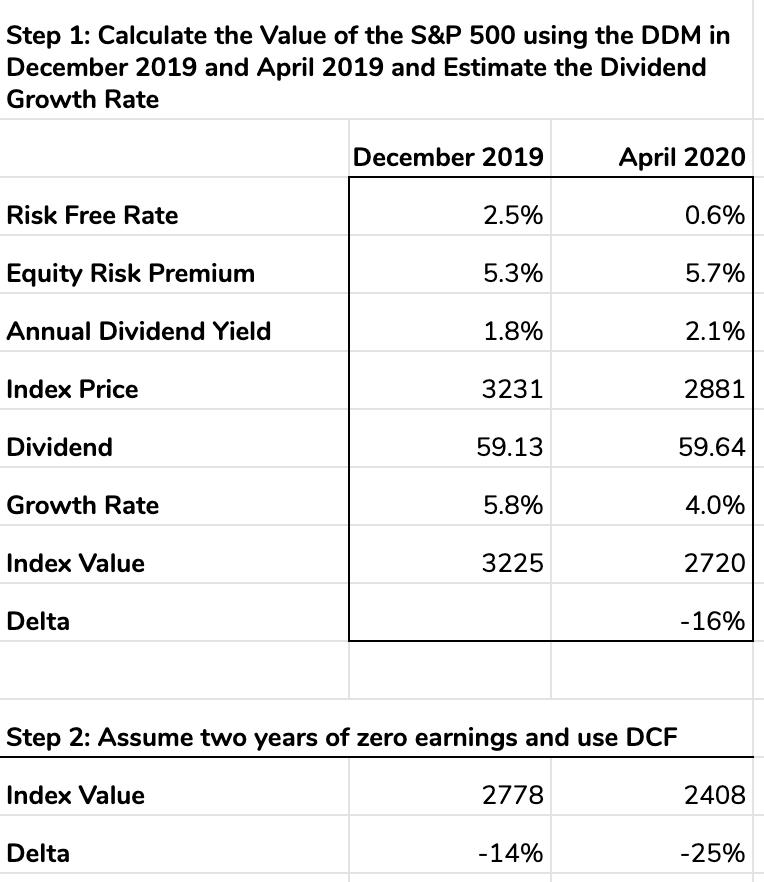# Why is the Stock Market Only Down 15%?

Why is the S&P index “only” down about 15%? Given all the economic turmoil, shouldn’t the index collapse? I was wondering this so I went to the source of all truth on the internet, Reddit. As I read the investing subreddit, I stumbled onto an explanation written by VPride1995 using a simple mental model that illuminated one potential answer.

The discount dividend model states that the value of a stock is the discounted cash flow of all future dividends. Companies pay a dividend to shareholders from profits. Public companies dividend out 30-40% of earnings on average.

The model says that the value of a stock is equal to next year’s dividends divided by (the cost of capital minus the dividend growth rate). Written as an equation

`Value = (Dividend_this_year x (1+dividend_growth_rate)) / (risk_free_rate + equity_risk_premium - dividend_growth_rate)`We can treat the S&P500 as one stock, even though it’s a basket of 500. We can plug in last year’s numbers in the table linked here to get an approximation for the value of the S&P 500 on Dec 31, 2019, of 3224, and this implies an S&P dividend growth rate of 5.8%. The S&P500 levels in April suggest that the market believes dividends will grow at 4.0%, down 30%.

But a lot has happened since then. If we assume two years of zero dividends, then dividends resume at their 2019 levels in 2022, then the value of the S&P500 is modeled to be 2777, which is just about a 15% decline. So you could explain the 15% decline by saying: the market has priced in two years of no dividends.

Alternatively and more pessimistically, if you assume the dividend growth rate falls from 5.8% to 4% after two years at zero dividends, then you should expect the index to fall 25% in value. Even in the extreme scenarios of no profits/dividends for two years and a 30% reduction in growth rate two years hence, the index should fall about 25%.

Like all mental models, this one is a gross simplification. It doesn’t consider the equity value or the appreciation of the S&P 500 during that time. And in reality, the stock market is a far more complex beast. There are major forces shaping the markets: the rise of passive investing, variances in trading volumes (which increase volatility), and vacillating dollar investment flows entering and exiting the US stock markets.

The DDM is not a unifying theory of economics, just a toy gyroscope to understand one principle. The model helped me understand why my intuition for stock market drops may not align well with how I ought to be thinking about them. Just because revenues fall or growth rates fall or profits fall a certain amount doesn’t mean the market should move in tandem with them.